Maths
Study Material

# Matrices Class 12-Introduction

In the digital era, every piece of information being processed is stored in the form of matrices. Let us get started with the fundamentals of matrix algebra through this lecture.

7 minutes long
Posted by Tanwir Silar, 28/7/2021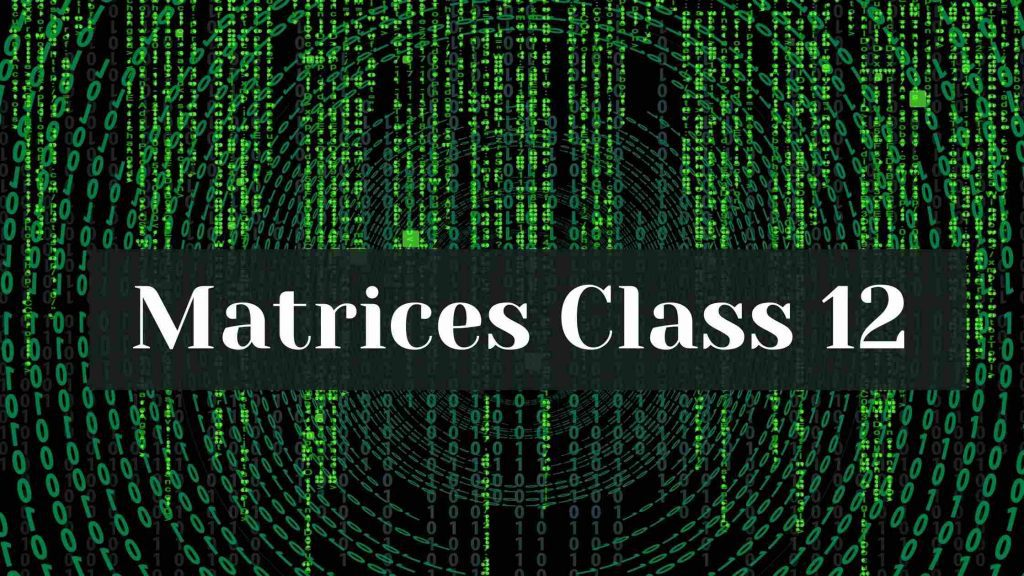Hesap Oluştur

Got stuck on homework? Get your step-by-step solutions from real tutors in minutes! 24/7. Unlimited.

A matrix is an ordered rectangular array of number or functions. The numbers or functions are called the elements or the entries of the matrix. In the real world, matrices are used to store and transform information. Therefore, we will read about matrices (class 12).

## Order of a matrix:

Matrices are usually denoted by capital letters. Any horizontal line of elements is referred to as a row. Any vertical line of elements is referred to as a column. A particular element of a matrix Amxn can be represented by the notation Aij where i is the number of the row and j is the number of the column where the element is present. For example, you can refer the image below:

## Types of Matrices

1. Column matrix:
A column matrix contains only one column..
For example: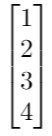is a column matrix of order 4 x 1.

2. Row matrix:
A matrix is a row matrix if it has only one row.
For example: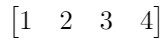is a row matrix of order 1 x 4.

3. Square matrix:
A square matrix of order n has equal number of rows and columns.
For Example: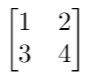is a square matrix of order 2.

4. Diagonal matrix:
A square matrix C is said to be a diagonal matrix if all of its non diagonal elements are zero, For example, if C = [cij]mxm is said to be diagonal matrix if cij = 0 if i ≠ j.
For Example: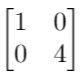is a diagonal matrix of order 2,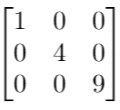is a diagonal matrix of order 3.

5. Scalar matrix:
When all the diagonal elements of a diagonal elements are equal, then it is a scalar matrix. Mathematically saying, a matrix B = [bij]mxm is said to be a scalar matrix, if bij = 0 if i ≠ j and bij = k, when i = j, for some constant k.
For Example: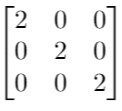is a scalar matrix of order 3.5.

6. Identity matrix:
If in a scalar matrix of order n all the diagonal elements are 1, then it is an Identity matrix or order n.
For Example: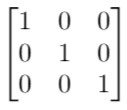is the identity matrix of of order 3,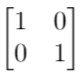is the identity matrix of of order 2.

7. Zero matrix:
A matrix is said to be zero matrix or null matrix if all its elements are zero. It is denoted by O. Hence, the order of the zero matrix, in an equation, depends on the matrices it is associated with.
For Example: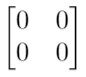,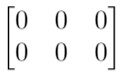are few examples of zero matrices.

## Equality of matrices

Two conditions determine if two matrices A = [aij] and B = [bij] are equal or not. They are:

1. the matrices are of the same order
2. each element of A is equal to the corresponding element of B , i.e., aij = bij for all i and j.

## Operations on matrices (class 12)

We can add any two matrices whose order is the same. Such as, the elements of the matrix C, where C= A + B, are the sum of the corresponding elements in A and B.
For example, two matrices A = [aij]mxn, B = [bij]mxn , the resultant sum C = A + B is given by [ cij ]mxn=
[ aij + bij ]mxn.

• Multiplication of matrix by scalar
Multiplying a matrix with a scalar k subsequently results in multiplying all the elements of a matrix with k.
For example, If A = [aij]mxn and k is a scalar, then kA = [k*aij]mxn

• Difference of matrices
Only matrices with the same order are compatible for difference operation. For example, The difference A – B can be rewritten as A + (-1) * B, i.e., adding A to (-1)* B, where (-1) * B is the scalar multiplication of B with -1.

• Multiplication of matrices
Two matrices A = [aik]mxn and B = [bkj]nxp are compatible for multiplication if the number of columns of A is equal to the number of rows of B, which in this case is equal to n. The resultant matrix C = AB has the order m x p.
The elements of C are calculated as follows: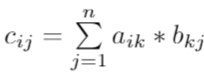. In this case, ith row elements of A are multiplied to jth column elements of B and added together to give us cij.
For Example:
Let us try to multiply two matrices A and B of order 3 x 2 and 2 x 3.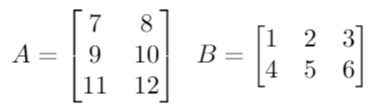Furthermore, We need to check the compatibility first. So then, it is clear, as the number of columns of A = 2 = number of rows of B.
The steps follow as we calculate cij for every i and j possible.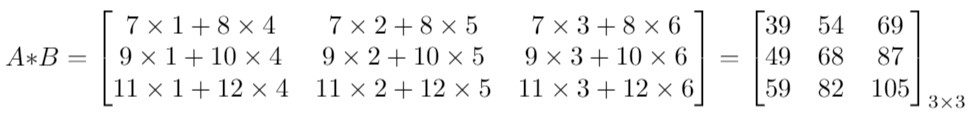It is evident that the resultant matrix C = AB has the order m x p = 3 x 3 in this case.

Above all, we hope this blog (Matrices-class 12) was helpful.